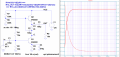#### nn_in

Joined Apr 14, 2009
14
How using constant current or active loads used in audio amps in diff amps and common emitter mode enables higher gain?

How is it better than using a resistor?

Any videos or actual experiments folks can share to explain this better.

Thanks.

#### crutschow

Joined Mar 14, 2008
27,734
How is it better than using a resistor?
A constant-current load for the collector of a transistor has a very high impedance, much higher than a resistor for the same collector bias current, which thus makes the gain higher.

•Kjeldgaard

#### LvW

Joined Jun 13, 2013
1,300
The key word is: Dynamic (differential) resistance.
* For an ohmic resistor the STATIC resistance R is equal to the DYNAMIC resistance r (effective for small voltage/current changes only).
This property results from the linear current-voltage relation.
Therefore, in order not to have a very large DC drop across the collector resistance, the ohmic value is limited to some kOhms (50kOhm would produce 50 volts for Ic=1mA).
* However, for an active load with a non-linear characteristic (like the C-E path of another transistor) the DC drop Vce can be limited to some volts only and - at the same time - the DYNAMIC resistance rce (1/h22), which is responsible for the voltage gain of the stage can be made much larger (for example rce=50 kohms).

#### nn_in

Joined Apr 14, 2009
14
Is this correct . For a CE gain is Rc/Re or Rc/Re' (if no Re is used) .Hence the gain is higher with constant current source?
So the Bjt rce adds up with the external constant current source rce?

#### nn_in

Joined Apr 14, 2009
14
Thanks. I had actually seen this too.

#### LvW

Joined Jun 13, 2013
1,300
Is this correct . For a CE gain is Rc/Re or Rc/Re' (if no Re is used) .Hence the gain is higher with constant current source?
So the Bjt rce adds up with the external constant current source rce?
No - that is not "correct". Please note, that in electronics no formula is really "correct". Everything is just an approximation because of some simplifications.
That means: We are using formulas and values which are good enough with respect to other uncertainties (tolerances, temperatur influences, parasitics,...).
For a common emitter stage (with RE feedback for signals to be amplified), the following gain expression is normally used:

G= - (Rc||rce1)/[(1/gm) + RE]
with coll. resistor Rc, output resistance of the main transistor rce1, transconductance gm.

The ohmic coll. resistor can be replaced by rce2 of another transistor:
G= - (rce1||rce2)/[(1/gm)+RE].

If - in addition - the simplificaiton RE>>1/gm=re´ is allowed, we get
G= - (rce1||rce2)/RE.

Last edited:

#### Bordodynov

Joined May 20, 2015
2,906

#### nn_in

Joined Apr 14, 2009
14
Thank You .
So Q2 is the active load?but Q2 is also receiving a input signal?

#### Bordodynov

Joined May 20, 2015
2,906
Yes Q2 works as an emitter repeater. Its transfer coefficient is close to one. As a result, the current (the variable part but not the constant part) through the resistor is small because it is affected by a low AC voltage. As a result, for the first transistor, it acts as a large-value resistor, the second pin of which is grounded in terms of alternating current.
The equivalent load of the first transistor is equal to the parallel connection of the base resistors of this equivalent resistor and the load recalculated to the repeater input (RLoad*(1+Beta_ekv)).